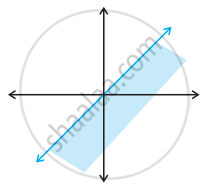# State whether the following statement is True or False. Solution set of x – y ≤ 0 is - Mathematics

MCQ
True or False

State whether the following statement is True or False.

Solution set of x – y ≤ 0 is• True

• False

#### Solution

This statement is False.

Explanation:

Because (1, 0) does not satisfy the given inequality and it is a point in shaded portion.

Concept: Algebraic Solutions of Linear Inequalities in One Variable and Their Graphical Representation
Is there an error in this question or solution?

#### APPEARS IN

NCERT Mathematics Exemplar Class 11
Chapter 6 Linear Inequalities
Solved Examples | Q 14 (vii) | Page 105
Share